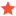APT CLS files in RoboDK - tool orientationLu.AFJunior MemberPosts: 2 Threads: 2 Joined: Jul 2019 Reputation: 0 09-11-2020, 10:59 AM Hi, I'm working with a .cls files from NX y I would like to understand how RoboDK do the translation of each points to targets. In this CLS file I only have xyz ijk, how RoboDK convert this to Euler angles? Because I need two vectors to define the Euler angles, does RDK assume that the second vector is paralalel to X/Y axis or is calculated from actual point to next point? Thanks, Find ReplyAlbertModeratorPosts: 927 Threads: 1 Joined: Apr 2018 Reputation: 45 09-14-2020, 12:16 PM RoboDK takes the ijk vector from the CLS file. This vector defines the tool axis. Including position, this gives you a 5-axis constrain. RoboDK automatically calculates the full orientation (pose) for each point, so when you generate a program it gives you Euler angles. Find Reply